﻿ New issues - Известия Казанского государственного архитектурно-строительного университета.Включен в Перечень ведущих рецензируемых научных журналов и изданий, определяемый ВАК## New issues

 First name, Middle name, Last name, Scientific degree, Scientific rank, Current position. Full and brief name of the organization, The organization address. Bakushev Sergey Vasilevish doctor of technical sciences, professor E-mail: This e-mail address is being protected from spambots. You need JavaScript enabled to view it Penza State University of Architecture and Construction The organization address: 440028, Russia, Penza, Germana Titova st., 28 Title of the article Differential equations of equilibrium for continuous media calculating with the approximation of volumetric and shear deformation diagrams by biquadrates functions (centrally symmetric deformation) Abstract. Problem statement. The purpose of the study is to derive differential equilibrium equations for a geometrically and physically nonlinear continuous medium under conditions of centrally symmetric deformation when the diagrams of volumetric and shear deformation are approximated by quadratic functions. Two mathematical models are used that describe the mechanical behavior of a material of a continuous medium: a model that does not take into account geometric nonlinearity and a model considering geometric nonlinearity. Results. The formation of physical dependencies is based on the calculation of the secant moduli of volumetric and shear deformation. When approximating the graphs of the volumetric and shear deformation diagrams using two segments of parabolas, the secant shear modulus in the first segment represents a linear function of the intensity of shear deformations; the secant modulus of volumetric expansion-contraction represents a linear function of the first invariant of the strain tensor. In the second section of the diagrams of both volumetric and shear deformation, the secant shear modulus is a fractional (rational) function of the intensity of shear deformations; the secant modulus of volumetric expansion-contraction is a fractional (rational) function of the first invariant of the strain tensor. Proceeding from the assumption that, generally speaking, the volumetric and shear deformation diagrams are independent on each other, six main cases of physical dependences are considered, depending on the relative position of the breakpoints of the volumetric and shear deformation diagrams graphs, each approximated by two parabolas. Based on the obtained physical equations, we derived differential equations of equilibrium in displacements for a continuous medium under conditions of centrally symmetric deformation. Conclusions. The significance of the results obtained for the construction industry lies in the fact that the obtained differential equations in displacements will make it possible to obtain a solution to the problems of calculating continuous media with central symmetry considering both geometry and load, the mechanical behavior of which is described taking into account physical and geometric nonlinearity, when approximation of diagrams plots of volumetric and shear deformation by biquadratic functions take place. Keywords. continuous medium, centrally symmetric deformation, approximation of volumetric and shear deformation diagrams, quadratic functions, differential equations of equilibrium in displacements, geometric linearity, geometric nonlinearity. For citations: Bakushev S.V. Differential equations of equilibrium for continuous media calculating with the approximation of volumetric and shear deformation diagrams by biquadrates functions (centrally symmetric deformation) // Izvestiya KGASU. 2020. №4(54) P.121-133.

New issues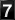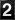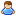Today 32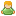Yesterday 55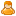This week 268Last week 341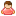This month 1166Last month 1293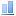All days 727727